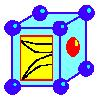#### by Krzysztof PARLINSKI

Last update: January 2, 2008

Phonon is a software (see list of Publications) for calculating phonon dispersion curves, and phonon density spectra of crystals, crystals with defects, surfaces, adsorbed atoms on surfaces, etc. from either a set of force constants, or from a set of Hellmann-Feynman forces calculated within an ab initio program (not included). One can use VASP, Wien2k, MedeA of Materials Design , Siesta, or other ab initio code which is able to optimize a supercell and calculate the Hellmann-Feynman forces. Phonon builds a crystal structure, using one of the 230 crystallographic space groups, finds the force constant from the Hellmann-Feynman forces, builds the dynamical matrix, diagonalizes it, and calculates the phonon dispersion relations, and their intensities. Phonon finds the polarization vectors, and the irreducible representations (Gamma point) of phonon modes, and calculates the total and partial phonon density of states. It plots the internal energy, free energy, entropy, heat capacity and tensor of mean square displacements (Debey-Waller factor). Phonon finds the dynamical structure factor for the coherent inelastic neutron scattering and the incoherent doubly differential scattering cross section for a single crystal and polycrystal. For polar cystals the LO/TO mode splitting can be included.